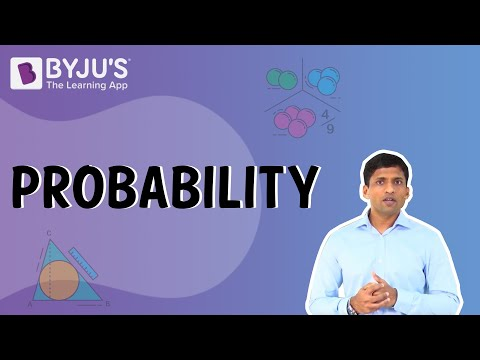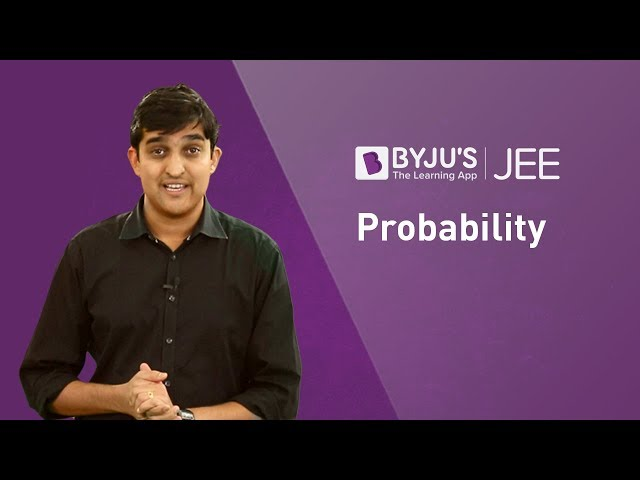# Probability Class 12 Notes Chapter 13

The sample space of an experiment of tossing three coins is S = {TTT, HHH, TTH, HHT, THT, HTH, HTT, THH}. Since the sample space comprises of 8 elements, therefore, the probability of occurring each sample point is 1/8. Let A and B be the events of displaying 2 heads and 1st coin showing tail respectively.

Then, A = {HHT, HHH, THH, HTH} and B = {THT, THH, TTT, TTH}.

Therefore P(A) = P({HHT}) + P ({HHH}) + P ({THH}) + P ({HTH})  =1/8 +1/8 +1/8 +1/8 =1/2.

Similarly, P(B) = P({THT}) + P({THH}) + P ({TTT}) + P ({TTH}) =1/8 +1/8 +1/8 +1/8 =1/2.

Also, A ∩ B = {THH} and P({THH}) = P(A ∩ B) = 1/8

The sample point of B which is favorable to event A is THH. Thus, P(A) considering B as the sample space (S) = 1/4. This P(A) is known as the conditional probability of A provided B has already occurred. The conditional probability of an event is denoted by P (A|B). Thus, from the above case P(A|B) = 1/4.

Or,

$$\begin{array}{l}P(A|B) = \frac{Total\;events\;favourable \;to \;A\cap B }{Total\;events \;favourable \;to \;B}\end{array}$$

Now, dividing both numerator and denominator by total elementary events of the S (sample space) we get:

$$\begin{array}{l}P(A|B) = \frac{\frac{n(A\cap B)}{nS}}{\frac{n(B)}{nS}}=\frac{P(A\cap B)}{P(F)}\end{array}$$

The above equation is valid only when P(A) ≠ 0. Hence, the conditional probability can be described as:

• 0 ≤ P (P|Q) ≤ 1
• P (P′|Q) = 1 – P (P|Q)
• P ((P ∪ Q)|R) = P (P|R) + P (Q|R) – P ((P ∩ Q)|R)
• P (P ∩ Q) = P (P) P (Q|P), P (P) ≠ 0
• P (P ∩ Q) = P (P) P (P|Q), P (Q) ≠ 0

If P and Q are independent, then

• P (P ∩ Q) = P (P) P (Q)
• P (P|Q) = P (P), P (Q) ≠ 0
• P (Q|P) = P (Q), P(P) ≠ 0Also Access NCERT Solutions for Class 12 Maths Chapter 13 NCERT Exemplar for Class 12 Maths Chapter 13

### Probability Class 12 Practice Questions

1. P and Q are two events such that P(P) ≠ 0. Find P(Q|P), if (i) P is a subset of Q (ii) P ∩ Q = φ
2. If a coin is tossed 9 times, find the probability of
1. A man speaks truth 4 out of 5 times. He throws a die and reports that it is a 3. Determine the probability that the number is actually 3.
2. Let P and Q be independent events with P(P) = 0.3 and P(Q) = 0.4. Find P(P ∩ Q), P(P ∪ Q), P (P|Q), P (Q|P).

 Bays Theorem Probability Distribution of Random Variable Total Probability Theorem Multiplication Rule of Probability

## Frequently Asked Questions on CBSE Class 12 Maths Notes Chapter 13 Probability

Q1

### What is conditional probability?

Conditional probability is defined as the likelihood of an event or outcome occurring, based on the occurrence of a previous event or outcome.

Q2

### What does statistics mean?

The science of collecting and studying data represented in the form of numbers is called as statistics.

Q3

### What is Bay’s theorem?

Bay’s Theorem is a mathematical formula for determining conditional probability.